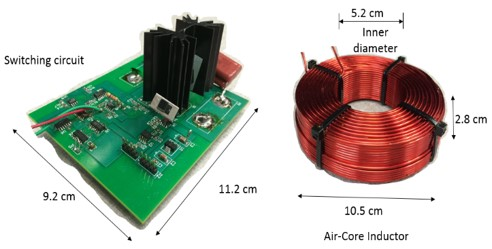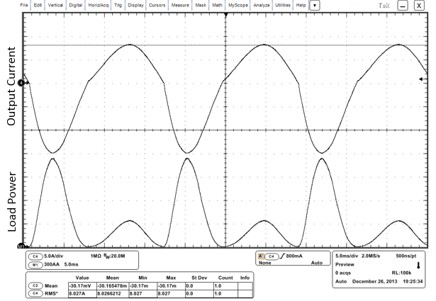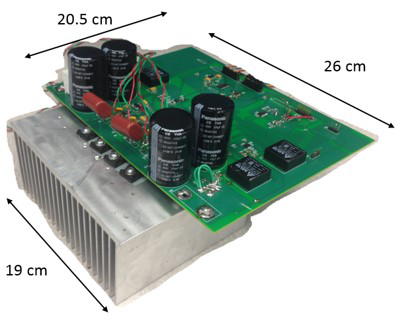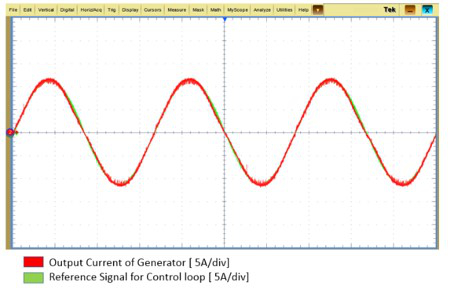LIBRARY

# Energy Harvesting from a Generator with Time-Dependent Output Inductance

Year: 2014Fig. 1. Hardware prototype of switching circuit of the generator and air-core inductor (together constitute the generator).
The output current of a generator with time-dependent output inductance is distorted because of non-linear inductance. The distortion reduces the harvested power from the generator. Reducing the distortion in the output current of the generator enhances the power output of the generator.

The conditions to maximize power obtained from a generator with time-dependent output inductance are derived mathematically in this paper. These conditions are realized using a converter behaving as variable impedance compensator. A hardware prototype of the converter is developed. The generator with time-dependent output inductance is designed and constructed in the lab using two discrete air-core inductors. An example of a generator with time-dependent output inductance is a magnetostrictive generator. The two inductance values for the designed generator are calculated to match the terminal behavior of the magnetostrictive generator. A picture of the developed generator is shown in Fig. 1. The output current (Fig. 2) for the 320-W generator shows a 29% THD (Total Harmonic Distortion). Reducing the distortion to 4% improves the power in the load to 465 W. Thus, even after accounting for the losses in the converter, there is 45% improvement in the harvested power from the generator. The experimental hardware of the variable impedance compensator is shown in Fig. 3, and the output current waveforms of the generator, on connecting the compensator are shown in Fig. 4.Fig. 2. Distorted output-current (5 A/div) from the generator and power (300 W/div) dissipated in the load without variable impedance compensator.Fig. 3. Hardware prototype of the variable impedance compensator.Fig. 4. Output current (5 A/div) of the generator with the variable impedance compensator.